Next: Random Velocity Up: Basic Equations for Radiative Previous: Basic Equations for Radiative   Contents

The basic equation for gas which is affected by the radiation is as follows: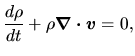(D.1)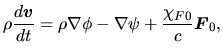(D.2)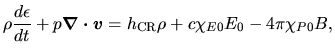(D.3)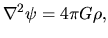(D.4)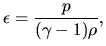(D.5)

where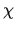,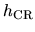, and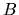are the absorption coefficient, the cosmic-ray heating rate perunit mass, and teh Planck function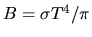. In equation (D.2), the term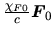represents the acceleration of gas due to the photon pressure. In equation (D.3),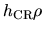,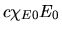and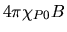represent, respectively the heating due to the CR particles, heating due to the absorption of radiation and cooling die to the emission. The frequence-integrated radiation energy density and radiation flux are defined as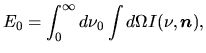(D.6)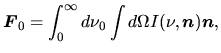(D.7)

where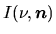denotes the specific intensity of radiation at frequency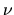along the direction vector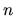. These equation could be solved if the the radiation transfer is solved. The frequency-averaged absorption coefficients are defined as follows: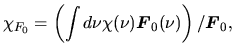(D.8)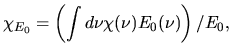(D.9)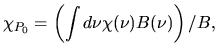(D.10)

While the equation for the radiation transfer is basically as follows: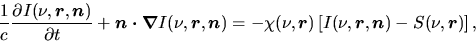(D.11)

where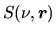represent the source term.Next: Random Velocity Up: Basic Equations for Radiative Previous: Basic Equations for Radiative   Contents
Kohji Tomisaka 2007-07-08